# How Many Workdays Left in Month / Year – Excel & Google Sheets

This tutorial will demonstrate how to find the number of workdays left in a Month or a Year in Excel and Google Sheets.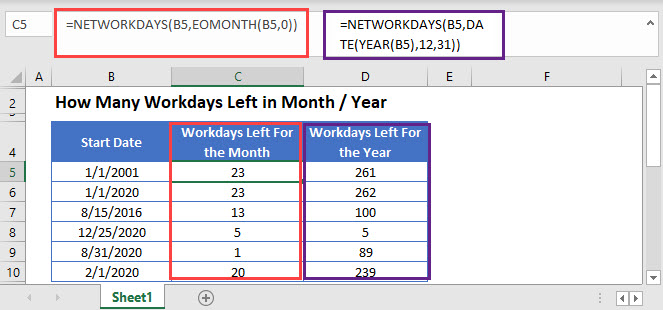<<master image>>

## NETWORKDAYS Function

The NETWORKDAYS Function calculates the number of workdays between two dates. Its syntax is: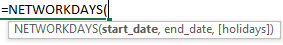We can use the NETWORKDAYS Function along with other functions to calculate the number of workdays left in a month or year.

## Workdays Left in Month

The EOMONTH Function returns the last day of a given month. It can be nested in the NETWORKDAYS Function to find the number of workdays left in a month like this:

``=NETWORKDAYS(B3,EOMONTH(B3,0))``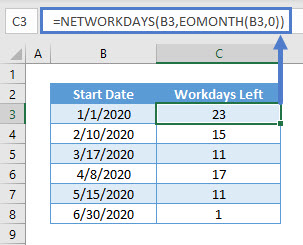Note: The NETWORKDAYS Function takes ‘Saturday’ and ‘Sunday’ as weekend. You can use the NETWORKDAYS.INTL Function to customise weekend days.

Workdays Left in Month from Today

If you want to find the number of workdays left in the current month starting with today, you can use the TODAY Function as the input date:

``=NETWORKDAYS(TODAY(),EOMONTH(TODAY(),0))``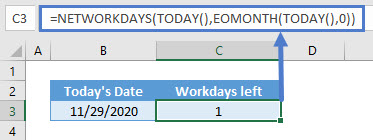Workdays Left in Year

To calculate the number of workdays left in a year, we can use the NETWORKDAYS Function:

``=NETWORKDAYS(B3,DATE(YEAR(B3),12,31))``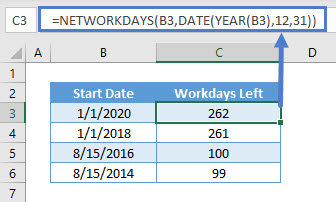Here we’ve defined the end date as the last day of the year, using the DATE and YEAR Functions.

Workdays left in Year from Today

To calculate the number of workdays left in the current year starting today, use TODAY as the start date:

``=NETWORKDAYS(TODAY(),DATE(B3,12,31))``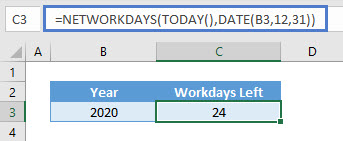## How Many Workdays Left in Month / Year in Google Sheets

This formula works exactly the same in Google Sheets as in Excel.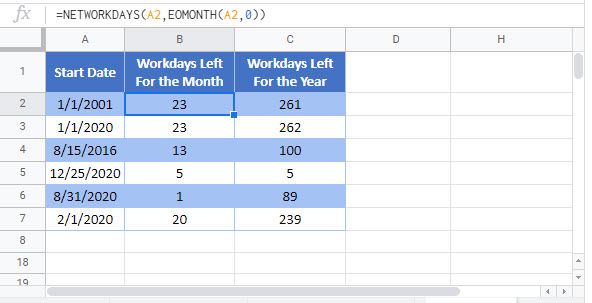### Excel Practice Worksheet

Practice Excel functions and formulas with our 100% free practice worksheets!